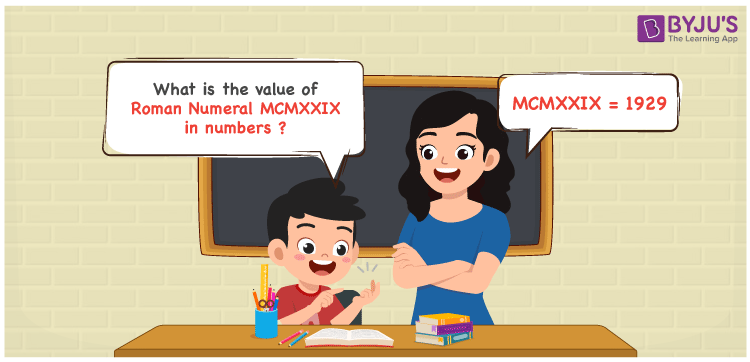Checkout JEE MAINS 2022 Question Paper Analysis : Checkout JEE MAINS 2022 Question Paper Analysis :

# MCMXXIX Roman Numerals

MCMXXIX Roman Numerals is 1929. Roman Numerals are a technique of representing numbers using the Roman alphabet. Download the Roman Numeral Conversion in PDF format to learn the simple technique of expressing Roman letters into numbers more effectively. In this article, we will learn how to depict the number form of MCMXXIX Roman Numerals in a detailed manner.

 Number Roman Numeral 1929 MCMXXIX

## How to Write MCMXXIX Roman Numerals?The following methods can be used to express the numerical value of MCMXXIX Roman Numerals

First Method:

• Break the Roman Numerals into single letters
• MCMXXIX = M + (M – C) + X + X + (X – I)
• Write the numerical value of each letter and add/subtract them
• MCMXXIX = 1000 + (1000 – 100) + 10 + 10 + (10 – 1) = 1929

Second Method:

In this method, we consider the groups of Roman Numerals for addition or subtraction

• MCMXXIX = M + CM + XX + IX = 1000 + 900 + 20 + 9 = 1929
• Therefore the numerical value of MCMXXIX Roman Numerals is 1929

## Video Lesson on Roman Numerals## Related Articles

Roman Numerals

Roman Numerals 1 to 50

Roman Numerals 1 to 100

Roman Numerals 1 to 1000

L Roman Numeral

Roman Numeral XXXIX

## Frequently Asked Questions on MCMXXIX Roman Numerals

### How do you write MCMXXIX Roman Numerals in numbers?

MCMXXIX Roman Numerals in numbers is written as 1929.

### Find the value of 1900 + 29 in Roman Numerals.

1900 + 29 = 1929. The number 1929 in Roman Numerals is MCMXXIX. Hence the value of 1900 + 29 in Roman Numerals is MCMXXIX.

### What is the difference between 1929 and 1800 in Roman Numerals?

1929 – 1800 = 129. The number 129 in Roman Numerals is CXXIX. Thus the difference between 1929 and 1800 in Roman Numerals is CXXIX.